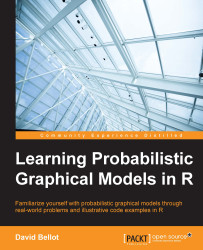•#### Learning Probabilistic Graphical Models in R#### Overview of this book

Probabilistic graphical models (PGM, also known as graphical models) are a marriage between probability theory and graph theory. Generally, PGMs use a graph-based representation. Two branches of graphical representations of distributions are commonly used, namely Bayesian networks and Markov networks. R has many packages to implement graphical models. We’ll start by showing you how to transform a classical statistical model into a modern PGM and then look at how to do exact inference in graphical models. Proceeding, we’ll introduce you to many modern R packages that will help you to perform inference on the models. We will then run a Bayesian linear regression and you’ll see the advantage of going probabilistic when you want to do prediction. Next, you’ll master using R packages and implementing its techniques. Finally, you’ll be presented with machine learning applications that have a direct impact in many fields. Here, we’ll cover clustering and the discovery of hidden information in big data, as well as two important methods, PCA and ICA, to reduce the size of big problems.
Learning Probabilistic Graphical Models in RCreditswww.PacktPub.comPrefaceFree Chapter
Probabilistic ReasoningExact InferenceLearning ParametersBayesian Modeling – Basic ModelsApproximate InferenceBayesian Modeling – Linear ModelsProbabilistic Mixture ModelsAppendixIndex## Summary

In this chapter, we saw the second (and presumably most successful) approach to performing Bayesian inference, with algorithms such as rejection and importance sampling, which are based on the use of a proposal distribution simpler than the one we want to estimate.

These two algorithms are usually efficient with low-dimensional cases but suffer from long convergence problems, when they converge at all, in high dimensions.

We then introduced the most important algorithm in Bayesian inference: the MCMC method using the Metropolis-Hastings algorithm. This algorithm is extremely versatile and has a nice property: it converges toward the distribution one wants to simulate. However, it needs careful tuning in order to converge, but its convergence is guaranteed in theory.

In the next chapter, we will explore the most standard statistical model ever: linear regression. While it seems beyond the scope of this book, this model is so important that it needs to be introduced. However, we will...